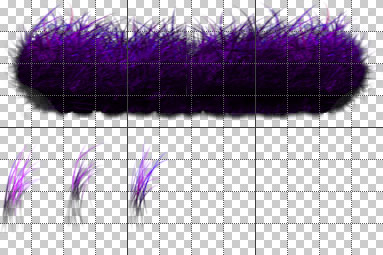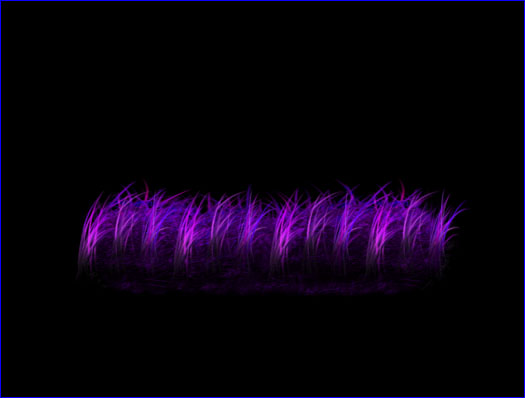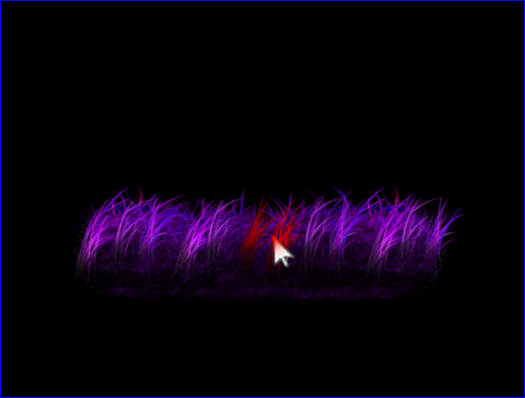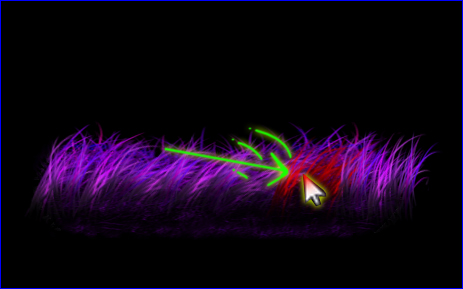## XNA Tutorial: Dynamic Grass(CLICK HERE TO GO TO UPDATED MONOGAME VERSION )

GOAL: Make grass move in response to a character's movement.

Step 1) Create your grass image(with at least 3 parts) and add it to Content in the solution explorer(.png or .dds). Maybe something like this:(note: I have gridlines and Info window turned on so I can check where the sprites will be in my texture image)

Step 2) New Project: (Windows Game 4.0) calling it Grass

Code:
```namespace Grass
{
public class Game1 : Microsoft.Xna.Framework.Game
{
// V A R S :
const int SCREENWIDTH = 800, SCREENHEIGHT = 600;
const bool FULLSCREEN = false;
GraphicsDeviceManager graphics;
SpriteBatch spriteBatch;
KeyboardState kb, old_kb; bool ignore_escape = false;
Texture2D texture;
DynamicGrass grass;

// C O N S T R U C T O R
public Game1()
{
graphics = new GraphicsDeviceManager(this) { PreferredBackBufferWidth = SCREENWIDTH, PreferredBackBufferHeight = SCREENHEIGHT, IsFullScreen = FULLSCREEN };
Content.RootDirectory = "Content";
}

//-------------
#region I N I T
//-------------
protected override void Initialize()
{
spriteBatch = new SpriteBatch(GraphicsDevice);

base.Initialize();
}
#endregion

//-------------
#region L O A D
//-------------
{
//grass = new DynamicGrass(GraphicsDevice, spriteBatch, texture);
}
protected override void UnloadContent() { }
#endregion

//-----------------
#region U P D A T E
//-----------------
protected override void Update(GameTime gameTime)
{
kb=Keyboard.GetState();
if ((!ignore_escape)&&(Keypress(Keys.Escape))) this.Exit();
ignore_escape=false;

base.Update(gameTime);
}
bool Keypress(Keys k) { if ((kb.IsKeyDown(k))&&(old_kb.IsKeyUp(k))) return true; return false; }
bool Keydown(Keys k) { if (kb.IsKeyDown(k)) return true; return false; }
#endregion

//-------------
#region D R A W
//-------------
protected override void Draw(GameTime gameTime)
{
GraphicsDevice.Clear(Color.Black);

old_kb = kb;
base.Draw(gameTime);
}
#endregion
}
}```

Step 4) There is no DynamicGrass class yet so right click in the solution explorer and add the "DynamicGrass" class and we'll start with the following:

Code:
```namespace Grass
{
class DynamicGrass
{
GraphicsDevice gpu;
int screenW, screenH;
SpriteBatch spriteBatch;
Texture2D texture;
Sprite bigGrass, gras1, gras2, gras3;
public Vector2 pos;

// C O N S T R U C T O R
public DynamicGrass(GraphicsDevice device, SpriteBatch _spriteBatch, Texture2D _texture)
{
spriteBatch = _spriteBatch;
texture = _texture;
gpu = device;
screenW = gpu.Viewport.Width;
screenH = gpu.Viewport.Height;

pos = new Vector2(screenW / 2 - 192, screenH / 2); //putting it near center of screen

//Init sprites - set the source Rectangle coordinates from the texture image (x,y,Width,Height)
bigGrass = new Sprite(new Rectangle(0, 0, 384, 128), pos);

//we don't care about the position of these yet - they will be used to create more grass later
Rectangle rect1 = new Rectangle(0, 128, 48, 128);
gras1 = new Sprite(rect1, pos); rect1.X += 64;    //get the sprite sheet coordinates
gras2 = new Sprite(rect1, pos); rect1.X += 64;    // "
gras3 = new Sprite(rect1, pos);                   // "
}

public void Draw(Vector2 position, float spacing)
{
int i=0;
pos = position;
spriteBatch.Draw(texture, pos, bigGrass.rect, Color.White);
float endPos = position.X + bigGrass.rect.Width;
do
{
if (i == 0) spriteBatch.Draw(texture, position, gras1.rect, Color.White);
else if (i == 1) spriteBatch.Draw(texture, position, gras2.rect, Color.White);
else if (i == 2) spriteBatch.Draw(texture, position, gras3.rect, Color.White);
position.X += spacing;
i++;
if (i>2) i=0;
} while (position.X < endPos);
}
}
}```

Note: You may find some things like Rectangle or Spritebatch underlined. If you right click on them and go into resolve - you should see a "using"...bla bla... if you click on that it will add the necessary "using" at the top of the class module to include access to the necessary namespace.

Step 5)We don't actually have a Sprite class yet, so let's add the following class:

Code:
```namespace Grass
{
class Sprite
{
public Rectangle rect; //image source
public Vector2 pos;    //position
public Vector2 vel;    //velocity
public float size;     //scale
public float rot;      //rotation
public float rot_vel;  //rotation velocity
public Vector2 origin; //origin (center point to rotate around)
public Color col;

// C O N S T R U C T O R  (only need to supply rectangle and position)
public Sprite(Rectangle rectangle, Vector2 position, float scale = 1f, float rotate = 0f)
{
rect = rectangle;
pos = position;
vel = Vector2.Zero;
size = scale;
rot = rotate;
rot_vel = 0f;
origin = new Vector2(rect.Width/2, rect.Height/2);
col = Color.White;
}
}
}```

Step 6) We'll want to be able to see the mouse for testing interaction. In Game1.cs in Initialize, add the following(before base.Initialize):
IsMouseVisible = true;
Also since we now have the classes we need you can uncomment the part in the Load function for creating the DynamicGrass instance:
grass = new DynamicGrass(GraphicsDevice, spriteBatch, texture);

Step 7) In Game1.cs, we will change Draw to the following:

Code:
```protected override void Draw(GameTime gameTime)
{
GraphicsDevice.Clear(Color.Black);

spriteBatch.Begin();
grass.Draw(grass.pos, 32);
spriteBatch.End();

old_kb = kb;
base.Draw(gameTime);
}```

Now if you run it, you should get something like this:Step 8) Now we'll want to keep track of the mouse states and where it was in the previous frame so we can get a velocity of impact. To do this first we need to add these variables at the top of Game1.cs:
MouseState ms, old_ms;
Vector2 mp, old_mp;

Step 9) Now in the Update of Game1.cs, we get the mouse data, mouse velocity, and pass it to an Update in DynamicGrass (which we haven't made yet). The code should look like this:
Code:
```protected override void Update(GameTime gameTime)
{
kb = Keyboard.GetState();
ms = Mouse.GetState(); mp = new Vector2(ms.X, ms.Y);
//---------------------------------

if ((!ignore_escape)&&(Keypress(Keys.Escape))) this.Exit();
ignore_escape=false;

Vector2 vel = mp - old_mp;
//----------------------------------

base.Update(gameTime);
}```
Note that we could place the grass anywhere instead of the default position(grass.pos).

Step 10: Change the draw function. Since Update now takes care of the draw position, we don't need to pass a position in draw and we also need to remember the old mouse data.

Code:
```protected override void Draw(GameTime gameTime)
{
GraphicsDevice.Clear(Color.Black);

spriteBatch.Begin();
grass.Draw(); //<-------changed-------------
spriteBatch.End();

old_kb = kb;
old_ms = ms; old_mp = mp; //<-----added-----
base.Draw(gameTime);
}```

Step 11) In DynamicGrass.cs -- we need to add some variables for keeping track of more detailed information of the grass bits. So let's add these:

Code:
```class DynamicGrass
{
GraphicsDevice gpu;
int screenW, screenH;
SpriteBatch spriteBatch;
Texture2D texture;
Rectangle bigGrass, gras1, gras2, gras3; //<-----changed
public Vector2 pos;
private static Random rnd;
Sprite[] grassBits;
int num_grass_bits;
public float spacing;
//--------------------------------```

Step 12) Now to change the constructor so that instead of using Sprites where unnecessary, we'll just use Rectangles to store the source rects and use Sprites for the grass bits which require this kind of detail. I randomize the rotation of each grass bit to create some variety.

Code:
```public DynamicGrass(GraphicsDevice device, SpriteBatch _spriteBatch, Texture2D _texture)
{
spriteBatch = _spriteBatch;
texture = _texture;
gpu = device;
screenW = gpu.Viewport.Width;
screenH = gpu.Viewport.Height;

pos = new Vector2(screenW / 2 - 192, screenH / 2); //putting it near center of screen

//-------------changed:
//Init sprites - set the source Rectangle coordinates from the texture image (x,y,Width,Height)
bigGrass = new Rectangle(0, 0, 384, 128);

Rectangle rect1 = new Rectangle(0, 128, 48, 128);
gras1 = rect1; rect1.X += 64;    //get the sprite sheet coordinates
gras2 = rect1; rect1.X += 64;    // "
gras3 = rect1;                   // "

rnd = new Random();
radius = rect1.Width/2; //might not be accurate
spacing = 32;
float endPos = bigGrass.Width;

num_grass_bits = bigGrass.Width / (int)spacing;
grassBits = new Sprite[num_grass_bits + 1];

int i=0;
Vector2 p = Vector2.Zero; //draw function will add final position to the offsets made in here...
Rectangle r;
do
{
int n=i%3; // goes: 0,1,2,0,1,2,0,1,2...
r=gras1; if (n==1) r=gras2; else if (n==2) r=gras3;
grassBits[i] = new Sprite(r, p, 1f, (float)rnd.NextDouble() * 0.2f);
grassBits[i].origin.Y = grassBits[i].rect.Height - 4; //rotate about bottom of grass bit
p.X += spacing;
i++;
} while (p.X < endPos);
}```

Step 13) Let's add an Update method to DynamicGrass.cs. It takes the difference between the mouse position and the position of approximately where a collision with a grass bit could occur and uses that difference and the circular radius of each combined to see if the circles overlap (meaning a collision occured). To test this - we just change the color to red for now. (Note this is why the bounding box region test is initially commented out - to ensure testing works):
Code:
```//-----------------
#region U P D A T E
//-----------------
public void Update(Vector2 grass_pos, Vector2 p, float col_rad, Vector2 vel) //grass_pos, collider_pos, collider_radius, collider_velocity
{
//if ((p.X < pos.X) || (p.X > pos.X + bigGrass.Width) || (p.Y < pos.Y) || (p.Y > pos.Y + bigGrass.Height)) return;

Vector2 diff;
pos = grass_pos;
int i = 0;
do
{
grass_pos = pos + grassBits[i].pos+grassBits[i].origin/2; //add the offset position of the big grass

diff = grass_pos - p;
distsqr = diff.LengthSquared();   //just leave it squared (avoid square root - is faster)
//Did the two circles overlap/collide?
{
grassBits[i].col = Color.Red;
}
else grassBits[i].col = Color.White;

i++;
} while (i < num_grass_bits);
}
#endregion```

Step 14) Modify the draw function. The positions (and eventually rotations and such) are made in the constructor and updates so now we simply draw the bits like so:
Code:
```public void Draw()
{
Vector2 grass_pos=pos;
spriteBatch.Draw(texture, pos, bigGrass, Color.White);
Sprite gb;
int i = 0;
do
{
gb = grassBits[i]; //just refer to it to reduce code size
grass_pos = pos + gb.pos + gb.origin;
spriteBatch.Draw(texture, grass_pos, gb.rect, gb.col, gb.rot, gb.origin, gb.size, SpriteEffects.None, 0);
i++;
} while (i<num_grass_bits);
}```

And now when you run the program you should see red collisions with mouse like so:We'll need to make several changes to the update function. We'll change the spacing to 16 to make the grass look thicker. We'll also add a grass_length = 35; to the constructor (approximating the length of the grass from origin to somewhere almost at the tip). This will rotate around the origin (in update) for each grass piece so we can test collision with a rotated version of the grass bit.

In Update we'll also test the rectangle of the whole thing to see if we can skip any unnecessary detection tests. What we'll do in each loop (for each piece of grass) is create an origin point (v1) and use grass length and .rot to calculate the rotated tip point (v2).

Then we check if the two circles bounding the tip and mouse are overlapping and if they are we apply a rotation in the approximate direction of the mouse's velocity(vel) and scale it down with some huge number (ie: /300 [depending on how sensitive you want the response to be]).

Then we apply a dampener to the velocity that makes itself 80% of what it was in the previous frame and we reduce the grass rotation by 95% each from so it sort of springs back to its starting position. A lot of changes are required(mostly in Update) but I put comments in the code to help you. Here's the entire new DynamicGrass class:

Code:
```namespace Grass
{
class DynamicGrass
{
//PRIVATE FIELDS:
//private static Random rnd; //(not using right now)
GraphicsDevice gpu;
SpriteBatch spriteBatch;
Texture2D texture;       // sprite sheet
int screenW, screenH;    // screen size
Rectangle bigGrass, gras1, gras2, gras3; // image sources
Sprite[] grassBits;      // grass pieces
int num_grass_bits;      // number of pieces
float grass_length;      // approximate length from grass origin to near grass tip

//PUBLIC FIELDS:
public Vector2 pos;      // top-left position of the grass group
public float spacing;    // space between each grass piece

//--------------------------------
#region C O N S T R U C T O R
//--------------------------------
public DynamicGrass(GraphicsDevice device, SpriteBatch _spriteBatch, Texture2D _texture)
{
spriteBatch = _spriteBatch;
texture = _texture;
gpu = device;
screenW = gpu.Viewport.Width;
screenH = gpu.Viewport.Height;

pos = new Vector2(screenW / 2 - 192, screenH / 2); //putting it near center of screen

//Init sprites - set the source Rectangle coordinates from the texture image (x,y,Width,Height)
bigGrass = new Rectangle(0, 0, 384, 128);

Rectangle rect1 = new Rectangle(0, 128, 48, 128);
gras1 = rect1; rect1.X += 64;    //get the sprite sheet coordinates
gras2 = rect1; rect1.X += 64;    // "
gras3 = rect1;                   // "

//rnd = new Random();   //(not using right now)
radius = rect1.Width/2; // might not be accurate
spacing = 16;           // 16 pixels should be good

num_grass_bits = bigGrass.Width / (int)spacing;
grassBits = new Sprite[num_grass_bits + 1];

int i=0;
Vector2 p = Vector2.Zero; //draw function will add final position to the offsets made in here...
float endPos = bigGrass.Width;
Rectangle r;
do
{
int n=i%3;
r=gras1; if (n==1) r=gras2; else if (n==2) r=gras3;
grassBits[i] = new Sprite(r, p, 1f, 0);
grassBits[i].origin.Y = grassBits[i].rect.Height/3*2; //rotate about bottom of grass bit
p.X += spacing;
i++;
} while (p.X < endPos);

grass_length = 35; //rough estimate of length
}
#endregion

//-----------------
#region U P D A T E
//-----------------
public void Update(Vector2 grass_pos, Vector2 collider_pos, float collider_radius, Vector2 vel)
{
bool check_collisions = true;
if ((collider_pos.X < pos.X) || (collider_pos.X > pos.X + bigGrass.Width) ||
(collider_pos.Y < pos.Y) || (collider_pos.Y > pos.Y + bigGrass.Height)) check_collisions = false;

Vector2 diff, v1, v2;
pos = grass_pos;
int i = 0;
do
{
grassBits[i].col = Color.White;
if (check_collisions)
{
// Step 1 - set up the vector points from origin(stem) to grass-tip(or close to it)
//          the collision circle to test collisions for is near the tip

//add the offset position of the big grass
v1 = pos + grassBits[i].pos + grassBits[i].origin; // v1 <--- origin

// Step 2 - find our where our tip actually is with the current rotation applied:
//          (Note: if you use something different than spriteBatch you may need to change these angle offsets)
float true_rot = grassBits[i].rot + MathHelper.PiOver2; //note: the tip is facing up so we need to add 90 degrees to point the vector correctly
v2.X = v1.X + grass_length * fCos(true_rot);
v2.Y = v1.Y - grass_length * fSin(true_rot);

// Step 3 - get the vector connecting the rotated tip and the collider_pos (in this case the mouse position)
diff = v2 - collider_pos;

// Step 4 - test to see if the distance between the centers is less than both radius distances added:
distsqr = diff.LengthSquared();   //just leave it squared (avoid square root - is faster)
if (distsqr <= radsqr) //<---Did the two circles overlap/collide? If yes:
{
grassBits[i].col = Color.Red;
// I just played with the division number until I had a response speed that looked good to me...
// The rotation velocity is set based on how fast the collider moves left or right (downward influence complicates things)
grassBits[i].rot_vel += vel.X / 300.0f; // <--adjust this(300) to boost or decrease collision sensitivity
}
}

// Step 5 - apply the rotation speed to the rotation amount
grassBits[i].rot += grassBits[i].rot_vel;

//put a limit on how far it can bend (and make it bounce off the ground if it happens)
if (grassBits[i].rot > 1.2f) {grassBits[i].rot = 1.2f; grassBits[i].rot_vel*=-1;}
if (grassBits[i].rot < -1.2f) { grassBits[i].rot = -1.2f; grassBits[i].rot_vel *= -1; }

// Step 6 - dampen the speed and try to spring back to starting angle
//each frame the rotation amount is only 95% of its previous value. This makes a nice tween to rotate back to 0 (which will point up)
grassBits[i].rot *= 0.95f;
//each frame we'll set the rotation speed at 80% of its previous speed.. makes a good decceleration curve.
grassBits[i].rot_vel *= 0.8f;

i++;
} while (i < num_grass_bits);
}
//helper classes and structs
public static float fCos(float angle) { return (float)Math.Cos((float)angle); }
public static float fSin(float angle) { return (float)Math.Sin((float)angle); }
#endregion

//-------------
#region D R A W
//-------------
public void Draw()
{
Vector2 grass_pos=pos;
spriteBatch.Draw(texture, pos, bigGrass, Color.White);
Sprite gb;
int i = 0;
do
{
gb = grassBits[i]; //just refer to it to reduce code size
grass_pos = pos + gb.pos + gb.origin;
spriteBatch.Draw(texture, grass_pos, gb.rect, gb.col, gb.rot, gb.origin, gb.size, SpriteEffects.None, 0);
i++;
} while (i<num_grass_bits);
}
#endregion
}
}```

And now if you run it you will see the grass moving in response to mouse collisions - like in this picture:Full Project Source